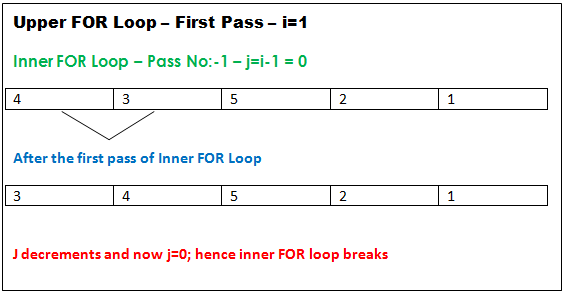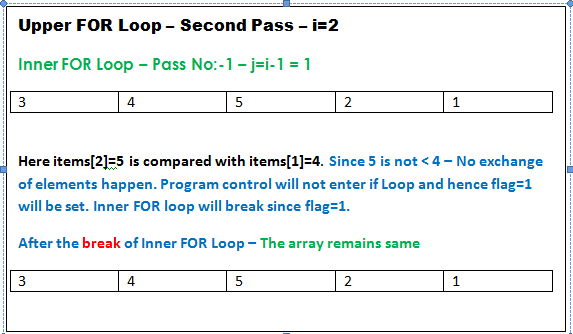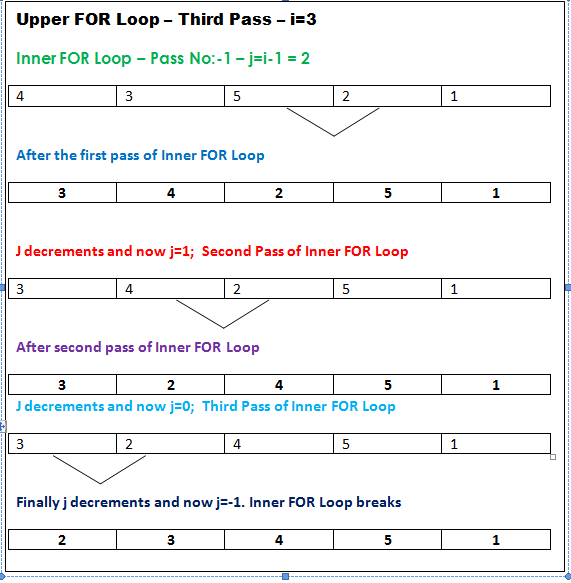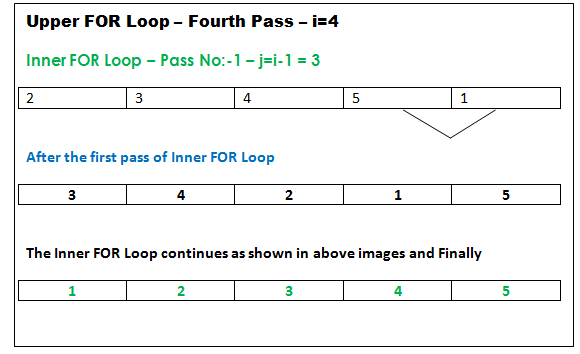## Audio

[Audio][slideshow]

So far we have seen 2 sorting algorithms:- 1) Bubble sorting and 2) Selection sorting. Now in this article, we are analyzing insertion sort algorithm with its example code suitable for C/C++/Java programming languages. I recommend you go through above articles of Bubble sorting and Selection sorting before reading further.

Insertion sorting algorithm sorts one element at a time. It begins by sorting the first 2 elements in order. In the next step, it takes the third element and compares it against the first two sorted elements. Exchanges are made if necessary and the 3 elements will be sorted with respect to each other. As next step, it takes the fourth element and it compares against the first 3 sorted elements. The process repeats until the whole array of elements are sorted.

Lets get into algorithm analysis using an example code snippet.

Note:- Since the algorithm is implemented with the help of 2 FOR loops only, it can be used as such for any programming languages like C/C++ or Java.

#### Example code for insertion sorting:-

`int items={4,3,5,2,1};int i,j,flag=0,temp;`

for(i=1;i=0&&flag==0;j--) // Inner FOR Loop
{

flag=1; // flag is set to 1 to break the inner loop, if it never enters if block statements.

if(items[j+1]

The working of the code snippet is explained using the pictures given below.Upper FOR Loop - First PassUpper FOR Loop-Second PassUpper FOR Loop - Third PassUpper FOR Loop-Fourth and Final Pass

#### Efficiency of insertion sorting:-

Efficiency of a sorting algorithm is determined using the number of comparisons it make while performing a sort. In the case of insertion sort, the number of comparisons highly depends on how the array is ordered initially. If the array is already sorted in initial condition, the number of comparisons made by insertion sort is n-1 (where n is the number of elements in the array). In the worst case scenario (where array is in the inverse order initially), insertion sort is just like Bubble sort and Selection sort. In the worst case, insertion sort makes comparisons of the order of n*n. So we can conclude that "insertion sorting" algorithm takes minimum time (when compared with Bubble and Selection sorting algorithms) if the array is already in order (or is nearly ordered).

[Lights][grids]